Courses
Courses for Kids
Free study material
Offline Centres
More

# Commutative Property of MultiplicationLast updated date: 24th Nov 2023
Total views: 147.9k
Views today: 2.47k## What is Multiplication?

Welcome to the world of magic – Maths Magic. Wondering how maths can be magic? To know that, you have to dive deep into the world of maths. To start with, let’s start with brushing up ‘what is multiplication?’ Multiplication is a process that will help you to understand the result of the combination of groups of equal size. It is also a process of shortening the repetitive addition. For example: 2 + 2 + 2 + 2 = 8 can be written as 2 × 4 = 8.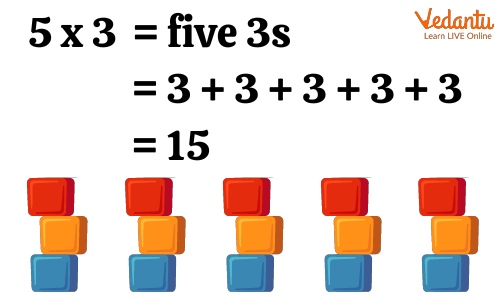There are six properties of multiplication: Closure property, Commutative property, Associative property, Distributive property, Multiplication by zero and Multiplicative identity. In this article, we will learn in brief about the Closure property, Associative property, Distributive property, Multiplication by zero and Multiplicative identity and in detail about the Commutative property. Let’s start.

## Closure Property of Multiplication

The Closure Property of Multiplication states that if two whole numbers a and b are multiplied, then their result will also be a whole number. In simple words, a × b will be the whole number for the whole numbers a and b.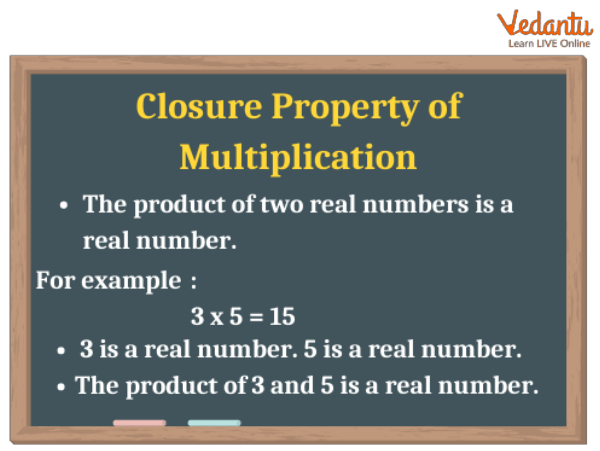Closure Property of Multiplication

## Associative Property of Multiplication

The result of the product of three or more numbers remains the same irrespective of their grouping. For example, (a × b) × c = a × (b × c).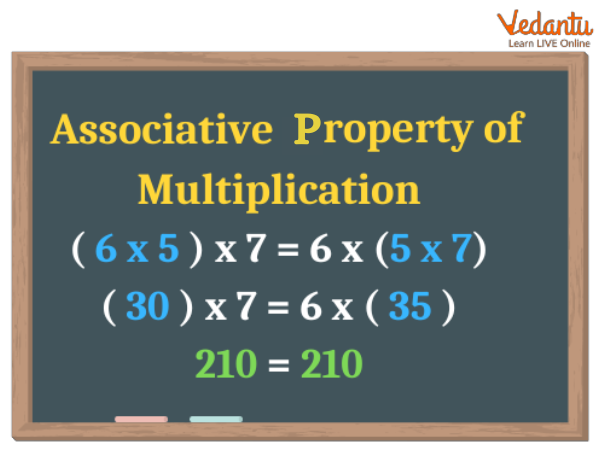Associative Property of Multiplication

## Distributive Property of Multiplication

The distributive property of multiplication states that if a, b and c are three numbers then,

a × (b + c) = (a × b) + (a × c).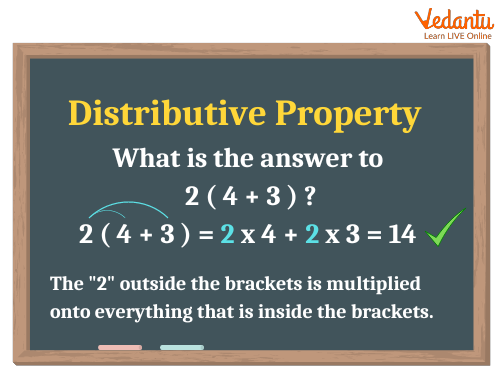Distributive Property of Multiplication

## Multiplication by 0

This is the most interesting property of multiplication. This property states that whenever any number is multiplied by 0, the result will be 0. In a simple way,

0 × anything = 0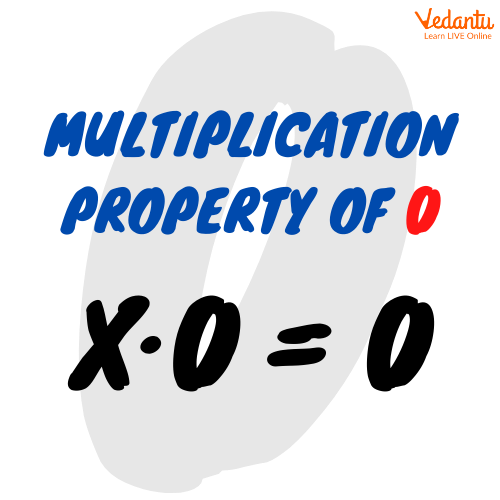Multiplication by 0

## Multiplicative Identity

The multiplicative identity states that if we multiply any number with 1, the answer is the number itself. In a simple way,

1 × a = a; 1 × b = b; 1 × c = c and so on.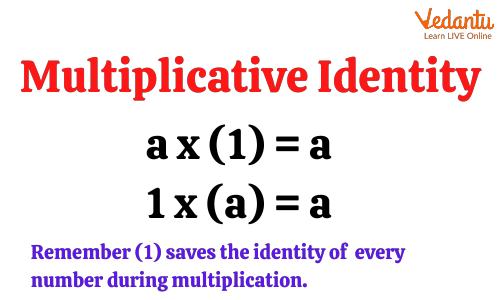Multiplicative Identity

## Commutative Property of Multiplication

The commutative property of multiplication states that changing the order of the numbers while multiplication does not change the result. In simpler words, whether you multiply a with b or b with a, the result will be the same. The order does not matter while multiplication. Example:

2 × 3 = 6 and

3 × 2 = 6

## Exercise on Commutative Property of Multiplication

Here are a few questions for you to solve:

1. 2 × _____ = 4 × _____ = 8

2. 3 × _____ = 5 × _____ = 15

3. 6 × _____ = 3 × _____ = 18

4. 5 × _____ = 7 × _____ = 35

5. 8× _____ = 6 × _____ = 48

6. 9 × _____ = 8 × _____ = 72

7. 4 × _____ = 5 × _____ = 20

8. 7 × _____ = 3 × _____ = 21

9. 5 × _____ = 9 × _____ = 45

10. 2 × _____ = 8 × _____ = 16

1. 2 × 4 = 4 × 2 = 8

2. 3 × 5 = 5 × 3 = 15

3. 6 × 3 = 3 × 6 = 18

4. 5 × 7 = 7 × 5 = 35

5. 8 × 6 = 6 × 8 = 48

6. 9 × 8 = 8 × 9 = 72

7. 4 × 5 = 5 × 4 = 20

8. 7 × 3 = 3 × 7 = 21

9. 5 × 9 = 9 × 5 = 45

10. 2 × 8 = 8 × 2 = 16

## Quick Facts on Commutative Property

 Use of commutative property started from around the end of 18th Century.The word ‘Commutative' is derived from the French word ‘Commute’ with adding a suffix of ‘ative’.The commutative property is applicable to only addition and multiplication. Subtraction and Division do not have commutative property.

Did you enjoy learning the properties of multiplication? You know, we have a lot more for you. You can explore other topics of mathematics as well. Don’t wait anymore. Explore now and enjoy your learning!

## FAQs on Commutative Property of Multiplication

1. Are all the arithmetic operations commutative?

No, only addition and multiplication have commutative properties. The other arithmetic operations are non-commutative i.e., changing their order changes the result.

2. How many properties are there for multiplication?

There are six properties of multiplication. They are

• Closure property,

• Commutative property,

• Associative property,

• Distributive property,

• Multiplication by zero and

• Multiplicative identity.